# Athletic club

All athletic club boys lined up by size. In front of Peter was one-eighth of the total. Right behind Peter stood his brother Radek and behind Radek another five-sixths of the total number of boys.

Mark the unknown total number of athletic club boys x.

1, Depending on x, express the number of boys who stood before Peter.
2, Depending on the quantity x, express the number of boys behind Peter.
3, Calculate the total number of athletic club boys.

n =  48

### Step-by-step explanation:

n/8+1+1+5/6n=n

n/8+1+1+5/6•n=n

n = 48

Our simple equation calculator calculates it.Did you find an error or inaccuracy? Feel free to write us. Thank you!Tips to related online calculators
Need help to calculate sum, simplify or multiply fractions? Try our fraction calculator.
Do you have a linear equation or system of equations and looking for its solution? Or do you have a quadratic equation?

## Related math problems and questions:

• Boys and girls 2The ratio of boys to girls in math club is 4:3 . After 8 more girls joined the Club, the ratio become 1:1. How many members are there in the club now?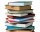Readers borrowed a total of 220 books in the library during the first three days. On the second day, readers borrowed half as many books as the first day and at the same time 20 fewer books than the third day. Depending on the quantity x, express the numb
• Unknown numberIf I reduced the sum of the numbers 70 and the unknown number three times, I would get 100. what is the unknown number?
• Unknown number 716% of the unknown number is by 21 less than unknown number itself. Determine the natural unknown number.
• Algebra problemThis is algebra. Let n represent an unknown number. 1. Eight more than the number n 2. Three times the number n 3. The product of the number n and eight 4. Three less than the number n 5. Three decreased by the number n
• Unknown number 5I think of an unknown number. If we enlarge it five times then subtract 3 and the result decreases by 75% we get one greater than the number. What number am I thinking of?
• UN 1If we add to an unknown number his quarter, we get 210. Identify unknown number.
• In the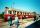In the 6th class, the average half-year mark in physics was 1.7. At the end of the year, Maťo improved his mark from 2 to 1, Ivanka from 3 to 2, and Elenka from 4 to 2. Pavlík worsened his mark from 3 to 4. The average mark of the whole class thus improve
• One dice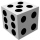Calculate the probability of one dice roll with the numbers 1, 2, 3, 4, 5, 6 on the walls. Write the results in a notebook in the shape of a fraction in the basic form: 2/3. a, The number 1 falls on the cube. b, The number 5 falls on the cube. c, An even
• What is 9What is the value of x in the proportion 2 and one-fourth over x = 1 and one-half over 3 and three-fifths? 2 and two-fifths 5 and two-fifths 8 and 1 over 10 12 and 3 over 20
• Unknown numberSamuel wrote unknown number. Then he had add 200000 to the number and the result multiply by three. When it calculated he was surprised, because the result would have received anyway, if write digit to the end of original number. Find unknown number.
• Expressions with variableThis is algebra. Let n represent an unknown number and write the following expressions: 1. 4 times the sum of 7 and the number x 2. 4 times 7 plus the number x 3. 7 less than the product of 4 and the number x 4. 7 times the quantity 4 more than the number
• Seven up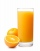Peter barman is making 8 gallons of Tropical trip punch. He has already poured in 1 3/4 gal of pineapple juice and 2 1/2 gal of orange juice. The only other ingredient us 7-Up. How much does 7-Up does Peter need?
• Trip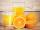On the trip drank 3/10 of pupils tea, 2/5 cola, 1/4 mineral water and remaining 3 juice. How many students were on the trip?
• Borrowing bicycle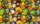Jakub lent a bicycle to friends who wanted to ride it. For a three-hour bike ride, Jakub received 2 chocolates. Yup. If you wanted a bike for 2 hours, you had to give Jakub 12 candies. Peter gave Jakub 1 chocolate and 3 candies. How long can Peter ride Ja
• Inheritance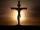After death father (♱ 62) remained mother (his wife) and its 3 children. Inheritance by law is that in the first mother will automatically get half of the property and other one-half inherited by heirs which are mother and her 3 children by the same share
• Unknown numberFind the unknown number equal to a quarter of a fifth of a number, which is by 152 more than an unknown number.